# Find the Most Frequent Numbers – Excel & Google Sheets

This tutorial will demonstrate how to find the frequencies of values in a dataset in Excel and Google Sheets.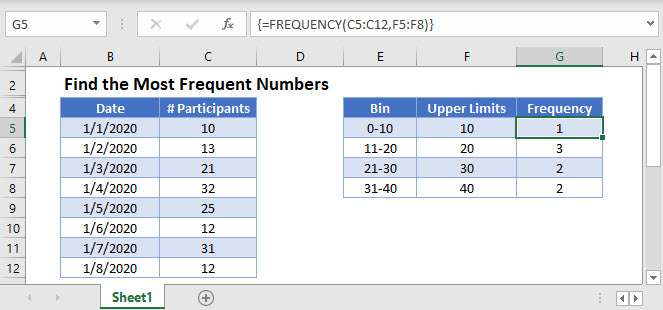## FREQUENCY Function

The FREQUENCY Function can be used to create a frequency table. Its syntax is: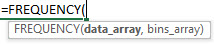The FREQUENCY Function takes a data range and a user-defined set of intervals and returns the number of occurrences for each interval.

``=FREQUENCY(C3:C27,F3:F6)``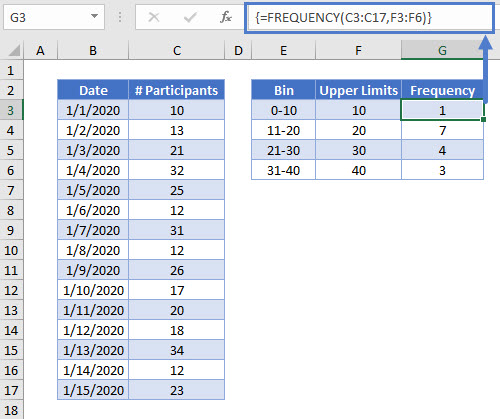First, divide the entire range of values into a series of intervals or “bins.” Our first bin includes Numbers of Participants between 0 and 10. For the FREQUENCY Function you only need the upper limit of each bin, so the input for the first bin is 10.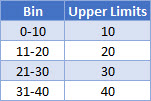Please note that this formula is an array formula, so if you are using Excel 2019 or earlier, you have to select the results range, enter the formula, and press CTRL + SHIFT + ENTER.

If you select one cell over the number of bin cells (as in the example shown above), you can get the number of occurrences that are outside your bins.

## Find the Most Frequent Numbers in Google Sheets

This formula works exactly the same in Google Sheets as in Excel.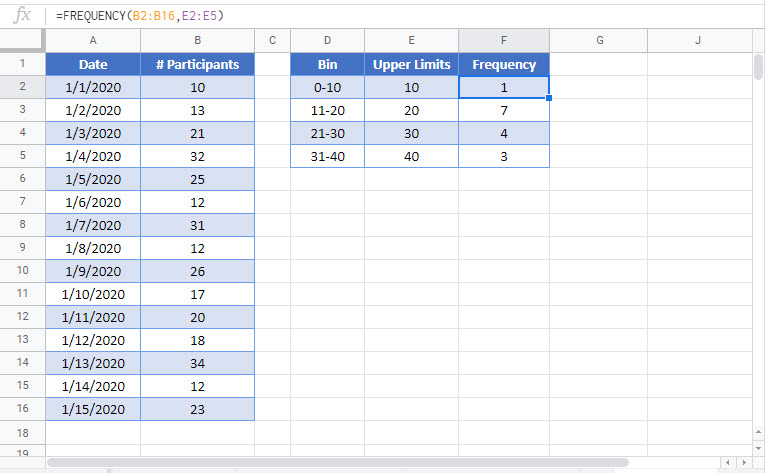### Excel Practice Worksheet

Practice Excel functions and formulas with our 100% free practice worksheets!